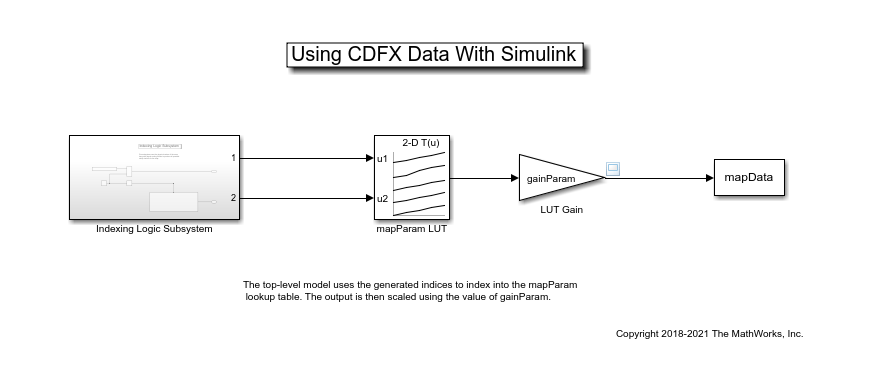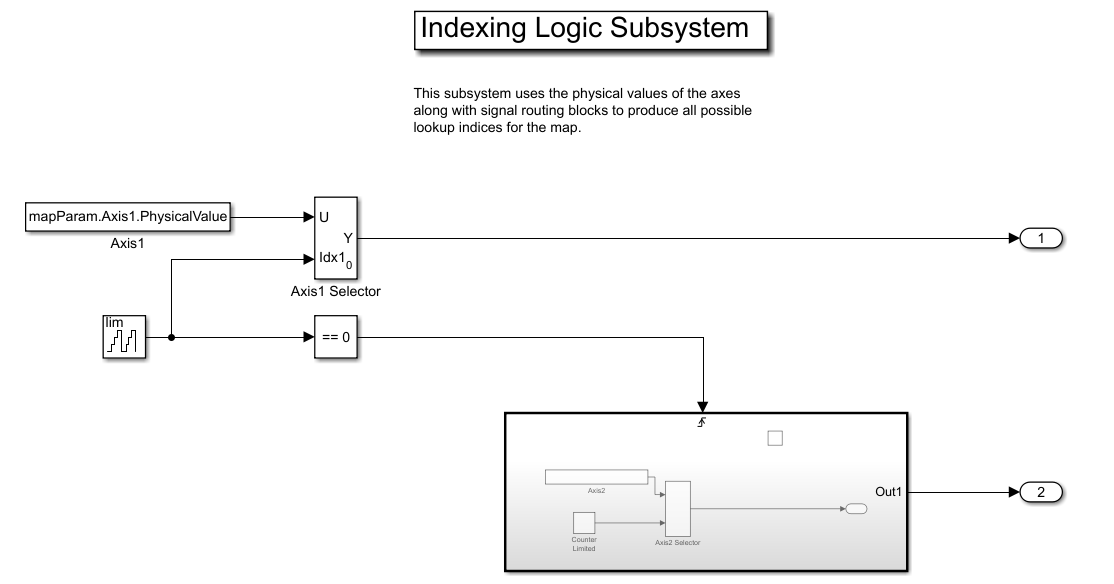This example shows how to use calibration data from a CDFX-file as inputs to a Simulink® model.

### Import Data

Import the calibration data using the `cdfx` function.

`cdfxObj = cdfx("CDFXExampleFile.cdfx")`
```cdfxObj = CDFX with properties: Name: "CDFXExampleFile.cdfx" Path: "/tmp/Bdoc23a_2213998_1547508/tpbdc9ff2c/vnt-ex88524458/CDFXExampleFile.cdfx" Version: "CDF20" ```

### Instantiate Local Variables

Use `getValue` to extract the desired parameters into the MATLAB® workspace.

`gainParam = getValue(cdfxObj, "ASAM.C.SCALAR.GAIN")`
```gainParam = 3 ```
`mapParam = getValue(cdfxObj, "ASAM.C.MAP")`
```mapParam = struct with fields: PhysicalValue: [5x5 double] Axis1: [1x1 struct] Axis2: [1x1 struct] ```

### Lookup-Gain Model

```open_system("CDFXSimulinkModel.slx"); cdfxMdl = gcs```
```cdfxMdl = 'CDFXSimulinkModel' ```This model contains:

• 2-D Lookup Table block to represent the `ASAM.C.MAP` parameter from the CDFX-file. The "Table data" field represents the physical value of the instance, and the "Breakpoint" fields represent the physical values of the axes.

• Gain block to represent the `ASAM.C.SCALAR.GAIN` parameter from the CDFX-file.

• To Workspace block to log the simulation data.

### Indexing Logic Subsystem

The Indexing Logic subsystem uses the physical values of the axes of the `ASAM.C.MAP` parameter, along with signal routing blocks and a triggered subsystem, to produce all valid combinations of lookup indices. This configuration can be useful if you need to test across the full range of possible input values of a calibration parameter.### Log Output Data in MATLAB

The output of the simulation is sent to MATLAB by the To Workspace block, where it is stored as a timeseries object called `mapData`. This data can now be inspected and visualized in the MATLAB workspace.

```sim(cdfxMdl); plot(mapData) title("Simulation Output for ASAM.C.MAP")```